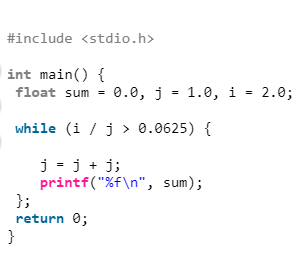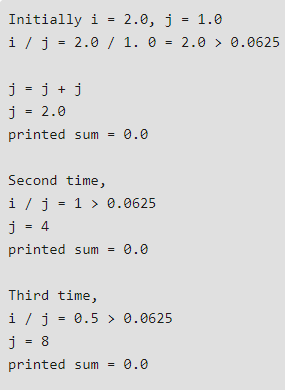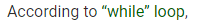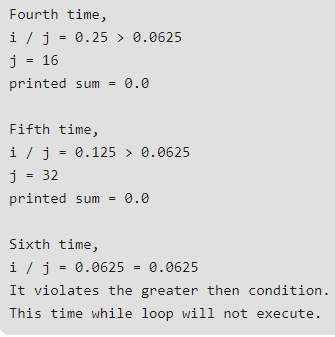# Gate CS-2019 Question Paper With Solutions

Q. 62 Consider the following C program:

``#include <stdio.h> ` ` ` `int` `main() { ` ` ``float` `sum = 0.0, j = 1.0, i = 2.0; ` `  ` ` ``while` `(i / j > 0.0625) { ` `     ` `    ``j = j + j; ` `    ``printf``(``"%f\n"``, sum); ` ` ``}; ` ` ``return` `0; ` `} ``

The number of times variable sum will be printed When the above program is executed is _________ .

Note: This was Numerical Type question.

(A) 5

(B) 6

(C) 4

(D) 0